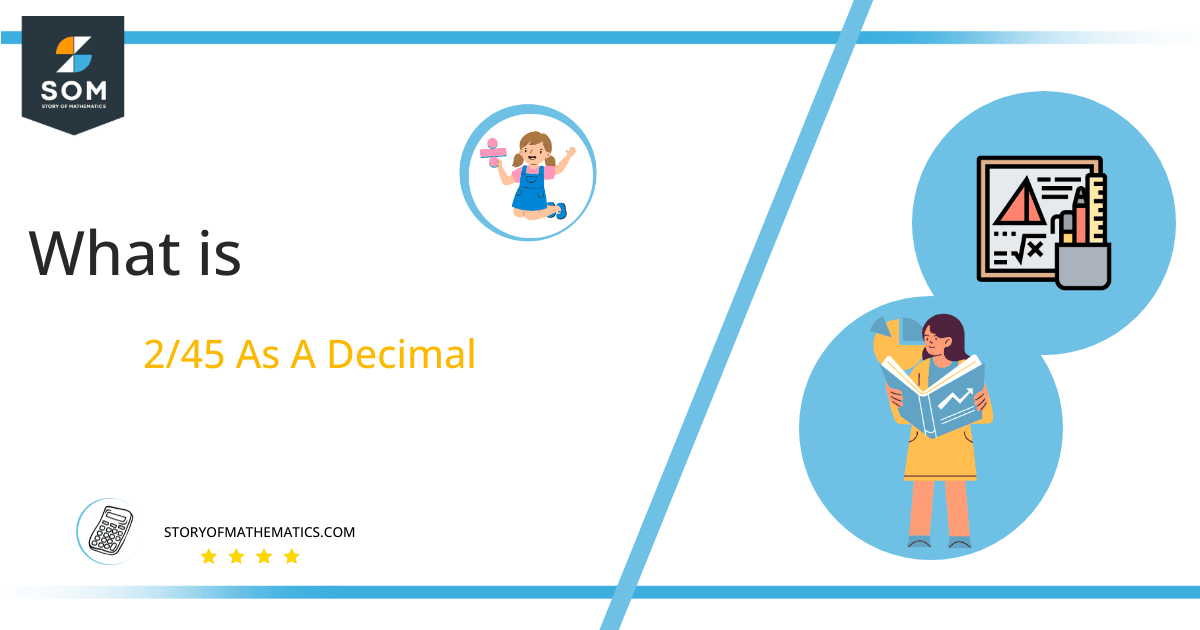# What Is 2/45 as a Decimal + Solution With Free Steps

The fraction 2/45 as a decimal is equal to 0.044.

We can represent the arithmetic operation of division in the form of fractions, which are numerals of the form p/q. Since fractions are an alternate expression of division, the division rules and procedures apply to them as well. There are several types of fractions such as proper, improper, mixed, etc. 2/45 is a proper fraction.

Here, we are more interested in the division types that result in a Decimal value, as this can be expressed as a Fraction. We see fractions as a way of showing two numbers having the operation of Division between them that result in a value that lies between two Integers.Now, we introduce the method used to solve said fraction to decimal conversion, called Long Division, which we will discuss in detail moving forward. So, let’s go through the Solution of fraction 2/45.

## Solution

First, we convert the fraction components, i.e., the numerator and the denominator, and transform them into the division constituents, i.e., the Dividend and the Divisor, respectively.

This can be done as follows:

Dividend = 2

Divisor = 45

Now, we introduce the most important quantity in our division process: the Quotient. The value represents the Solution to our division and can be expressed as having the following relationship with the Division constituents:

Quotient = Dividend $\div$ Divisor = 2 $\div$ 45

This is when we go through the Long Division solution to our problem.Figure 1

## 2/45 Long Division Method

We start solving a problem using the Long Division Method by first taking apart the division’s components and comparing them. As we have 2 and 45, we can see how 2 is Smaller than 45, and to solve this division, we require that 2 be Bigger than 45.

This is done by multiplying the dividend by 10 and checking whether it is bigger than the divisor or not. If so, we calculate the Multiple of the divisor closest to the dividend and subtract it from the Dividend. This produces the Remainder, which we then use as the dividend later.

However, in our case, 2 x 10 = 20, which is still smaller than 45. Therefore, we again multiply by 10 to get 20 x 10 = 200, which is larger than 45. To indicate the double multiplication, we add a decimal point “.” followed by a 0 to our quotient.

Now, we begin solving for our dividend 2, which after getting multiplied by 10 becomes 200.

We take this 200 and divide it by 45; this can be done as follows:

200 $\div$ 45 $\approx$ 4

Where:

45 x 4 = 180

This will lead to the generation of a Remainder equal to 200 – 180 = 20. Now this means we have to repeat the process by Converting the 20 into 200 and solving for that:

200 $\div$ 45 $\approx$ 4

Where:

45 x 4 = 180

This, therefore, produces another Remainder which is equal to 200 – 180 = 20. We now have the three decimal places for our quotient, so we stop the division process. Our final Quotient is 0.044 with a final remainder of 20.Images/mathematical drawings are created with GeoGebra.